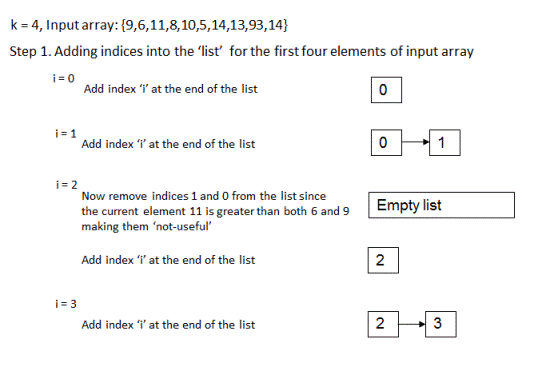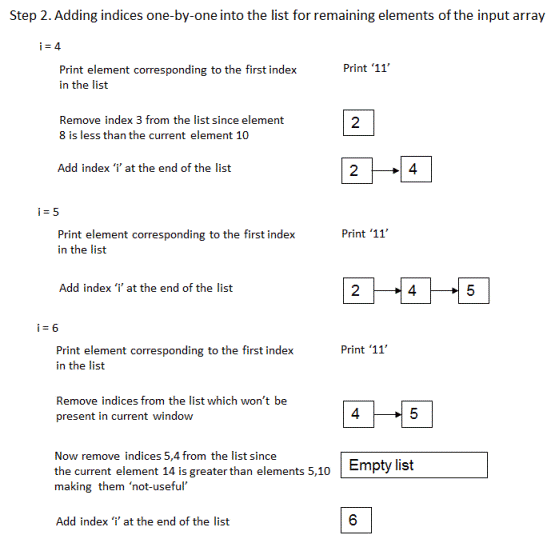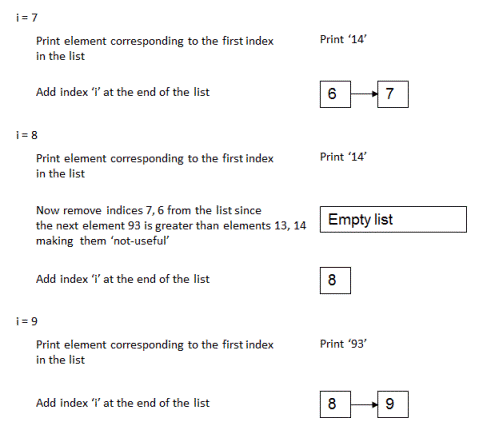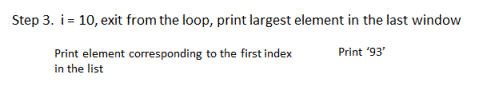# Find maximum element from each sub-array of size 'k'| Set 2

If you are given an integer array and an integer 'k' as input, write a program to print elements with maximum values from each possible sub-array (of given input array) of size 'k'. If the given input array is {9,6,11,8,10,5,14,13,93,14} and for k = 4, output should be 11,11,11,14,14,93,93. Please observe that 11 is the largest element in the first, second and third sub-arrays - {9,6,11,8}, {6,11,8,10} and {11,8,10,5}; 14 is the largest element for fourth and fifth sub-array and 93 is the largest element for remaining sub-arrays.

## Algorithm/Insights

In this previous post, we have looked at the solution for this same problem which makes use of self-balancing Binary Search Trees. In this post, we will look at an approach which makes use of Dequeue data structure to solve this problem in O(n) time. A Dequeue data structure is nothing but a linked list where you can add and delete nodes from both ends of the linked list.

Intuition: Let dequeue be denoted by the 'list'. This 'list' stores the indices of array elements in it(and not the values of array elements). We maintain the state of the 'list' in such a way that the array elements corresponding to the indices in the list are sorted in the decreasing order. We also make sure that the list does not contain the indices which are not part of current window of size 'k'. This way we are making sure that the element corresponding to first index in the list is the largest element in current window of size 'k'.

Now let's try to understand the steps of the algorithm.
1. For first 'k' elements of the input array that is for i = 0 up to i = k-1 :
(a). Before adding index 'i' into the 'list', we remove all indices 'j's from the rear end of the 'list' for which array[j] is less than array[i]. This is because the elements corresponding to these indices are never going to the largest elements for this sub-array of first 'k' elements as well as for any subsequent sub-array. Hereafter, we call such elements as 'not-useful' elements. We make use of the following 'while loop' for this step -
while (!list.isEmpty() && array[list.getLast()] < array[i])
{
list.removeLast();
}
(b). Now we add index 'i' at the end of the 'list'.

After execution of step #1a and #1b, 'list' contains indices corresponding to useful elements only and the first index in the list corresponds to the largest element from the first sub-array(window) of 'k' elements. In the below steps as well, we will maintain the same state of the list where the first index in the list corresponds to the largest element from the current window of 'k' elements and the 'list' contains indices for useful elements only.

2. Now for remaining elements of the input array that is for i = k up to i = n-1 (n: length of the input array) -
(a). We print the element corresponding to the first index in the 'list'. This element would be the largest element in the last seen window of 'k' elements.
(b). Now we remove all indices from the front-end of the 'list' which won't be included in the current window of size 'k'. Note that for this current window of size 'k', index of the last element would be 'i', index of the second last element would be 'i-1' and the index of the first element would be 'i-k+1'. Any indices present in the list which are less than 'i-k+1' won't be included in current window. For this step, we use the following 'while loop' -
while (!list.isEmpty() && (list.getFirst() < (i-k+1)))
{
list.removeFirst();
}
(c). Now very similar to step #1a, we remove all indices corresponding to 'not-useful' elements starting from the rear-end of the 'list'. This helps to maintain the indices in the order such that corresponding array elements are sorted in the decreasing order.
(d). We add index 'i' at the end of the 'list'.

3. After execution of step #2, the first index in the list now corresponds to the largest element in the last window of size 'k'. We print the element corresponding to this index which marks the completion of this algorithm.

Here is a step by step illustration of this algorithm for the input array as {9,6,11,8,10,5,14,13,93,14} and for k = 4,The time complexity of this algorithm is O(n) where 'n' is the length of the input array. Note that in this algorithm every element is compared at most twice for remove operation from the rear-end and every element on an average is compared only once for remove operation from the front-end of the 'list'. Therefore, for every element of the array there are constant number of operations that are performed on it making time complexity as O(n). The extra space used by this algorithm is O(k).

## Code Snippet

```
package com.ideserve.nilesh.questions;

/**
* <b>IDeserve <br>
* In O(nlogk) time, this code finds out maximum element from each sub-array of size 'k'.
* @author Nilesh
*/

public class MaximumfromEachSubarray
{
public MaximumfromEachSubarray()
{
}

private void printMax(int[] array, int low, int high)
{
int maxValue = Integer.MIN_VALUE;
for (int i = low; i <= high; i++)
{
if (array[i] > maxValue)
{
maxValue = array[i];
}
}

System.out.println(maxValue);
}

public void simplePrintMaxfromEachSubarray(int[] array, int k)
{
// {4,2,12,34,23,35,44,55};
int low = 0, high = low + k - 1;

while (high < array.length)
{
printMax(array, low, high);
low  += 1;
high += 1;
}
}

public void printMaxfromEachSubarray(int[] array, int k)
{

for (int i = 0; i < k; i++)
{
// remove all useless elements present at the front of the list
while (!list.isEmpty() && array[list.getLast()] < array[i])
{
list.removeLast();
}

// add index of current element at the back
}

for (int i = k; i < array.length; i++)
{
// first element present in the list is the greatest element for the last 'k' sized sub-array
System.out.println(array[list.getFirst()]);

// now remove all indices of elements from the list which do not belong to current window
while (!list.isEmpty() && (list.getFirst() < (i-k+1)))
{
list.removeFirst();
}

// now again remove all useless elements present at the front of the list
// remove all useless elements present at the front of the list
while (!list.isEmpty() && array[list.getLast()] < array[i])
{
list.removeLast();
}

// and finally insert this new element at the back of the list
}

// now print the largest element from the last sub-array(window)
System.out.println(array[list.getFirst()]);
}

public static void main(String[] args)
{
MaximumfromEachSubarray solution = new MaximumfromEachSubarray();

int[] array = {9,6,11,8,10,5,14,13,93,14};
int k = 4;

System.out.println("Maximum elements from each sub-array of specified size are - ");
solution.printMaxfromEachSubarray(array, k);
}
}
```

## Order of the Algorithm

Time Complexity is O(n)
Space Complexity is O(k)